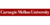# Probability & Statistics

## Probability & StatisticsCarnegie Mellon University

Need more information? Get more details on the site of the provider.

### Starting dates and places

There are no known starting dates for this product.
• View related products with starting dates: Statistics.

### Description

This course introduces students to the basic concepts and logic of statistical reasoning and gives the students introductory-level practical ability to choose, generate, and properly interpret appropriate descriptive and inferential methods. In addition, the course helps students gain an appreciation for the diverse applications of statistics and its relevance to their lives and fields of study. The course does not assume any prior knowledge in statistics and its only prerequisite is basic algebra.

We offer two versions of statistics, each with a different emphasis: Probability and Statistics and Statistical Reasoning. Each course includes all expository text, simulations, case studies, comp…

There are no frequently asked questions yet. If you have any more questions or need help, contact our customer service.

This course introduces students to the basic concepts and logic of statistical reasoning and gives the students introductory-level practical ability to choose, generate, and properly interpret appropriate descriptive and inferential methods. In addition, the course helps students gain an appreciation for the diverse applications of statistics and its relevance to their lives and fields of study. The course does not assume any prior knowledge in statistics and its only prerequisite is basic algebra.

We offer two versions of statistics, each with a different emphasis: Probability and Statistics and Statistical Reasoning. Each course includes all expository text, simulations, case studies, comprehension tests, interactive learning exercises, and the StatTutor labs. Each course contains all of the instructions for the four statistics packages options we support. To do the activities, you will need your own copy of Microsoft Excel, Minitab, the open source R software, TI calculator, or StatCrunch.

One of the main differences between the courses is the path through probability. Probability and Statistics includes the classical treatment of probability as it is in the earlier versions of the OLI Statistics course.

Topics Covered: Estimated Time to Complete Course: This course is designed to be equivalent to one semester of a college statistics course. Additional Software or Materials Required: To do the activities, you will need your own copy of Microsoft Excel, Minitab, the open source R software (free), TI calculator, or StatCrunch. You will also need to have Flash, Java, and mathml installed; these programs are free. More detailed information is provided in the course under “Test and Configure Your System.” Maintenance Fee (per student): Free for independent learners; \$25 for academic students.

## In-Depth Description

There are two versions of the OLI Statistics course: Probability and Statistics and Statistical Reasoning. Both versions have four units with the difference between the two versions being in the third unit (Probability) as outlined below.

Unit 1 Exploratory Data Analysis. This is organized into two modules – Examining Distributions and Examining Relationships. The general approach is to provide students with a framework that will help them choose the appropriate descriptive methods in various data analysis situations. Unit 2 Producing Data. This unit is organized into two modules – Sampling and Study Design. Unit 3 Probability. As stated above, this is the unit where the two versions of the course differ. In the Probability and Statistics course the unit is a classical treatment of probability and includes basic probability principles, conditional probability, discrete random variables (including the Binomial distribution) and continuous random variables (with emphasis on the normal distribution). The probability unit of the Statistical Reasoning version of the course essentially acts as a “bridge” to the inference section and includes only those concepts necessary to support a conceptual understanding of the role of probability as the “machinery” behind inference. Both probability parts culminate in a discussion of sampling distributions that is grounded in simulation. Unit 4 Inference. This unit introduces students to the logic as well as the technical side of the main forms of inference: point estimation, interval estimation and hypothesis testing. The unit covers inferential methods for the population mean and population proportion, Inferential methods for comparing the means of two groups and of more than two groups (ANOVA), the Chi-Square test for independence and linear regression. The unit reinforces the framework that the students were introduced to in the Exploratory Data Analysis for choosing the appropriate, in this case, inferential method in various data analysis scenarios.
Throughout the course there are many interactive elements. These include: simulations, “walk-throughs” that integrate voice and graphics to explain an example of a procedure or a difficult concept, and, most prominently, computer tutors in which students practice problem solving, with hints and immediate and targeted feedback. The most elaborate of such activities is the “StatTutor,” a tool that supports students as they go through the processes of data analysis while emphasizing the big picture of statistics.

The course is built around a series of carefully devised learning objectives that are independently assessed. Most of the interactive tutors are tagged by learning objective and skill, and so student work can be tracked by the system and reported to the instructor via the Learning Dashboard. These give the instructor insight into mastery of learning objectives and skills, both for the class as a whole and for individual students.

The course was designed to be used as a stand alone (with no instruction in the background) however studies have shown that it is best and most effectively used in the hybrid mode together with face to face instruction.

## StatTutor Exercises [Enter Course]

This course contains only the StatTutor lab exercises.

There are no reviews yet.